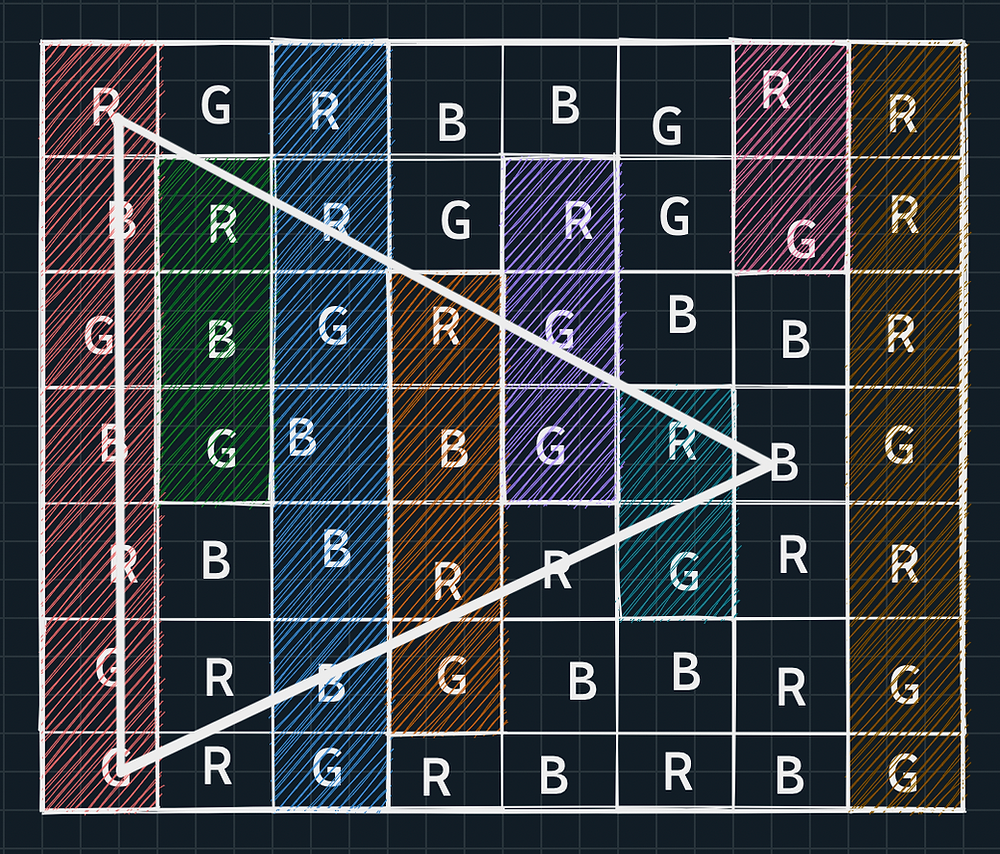Search

# Maximum Area of Triangle! InterviewBit Solution

### Problem Description:

Given a character matrix of size N x M in the form of a string array A of size N where A[i] denotes ith row.

Each character in the matrix consists of any one of the following three characters {'r', 'g', 'b'} where 'r' denotes red colour similarly 'g' denotes a green colour and 'b' denotes blue colour.

You have to find the area of the largest triangle that has one side parallel to the y-axis i.e vertical and the colour of all three vertices are different.

NOTE:

• If the area comes out to be a real number then return the ceil of that number.

### Problem Constraints:

```2 <= N, M <= 10^3
A[i][j] = 'r' or A[i][j] = 'g' or A[i][j] = 'b'```

### Input Format:

The first and only argument is a string array A of size N denoting the 2D character matrix.

### Output Format:

Return a single integer denoting the area of the largest triangle that has one side parallel to the y-axis i.e vertical and the colour of all three vertices are different.

If the area comes out to be a real number then return the ceil of that number.

### Example Input:

Input 1:

` A = ["rrrrr", "rrrrg", "rrrrr", "bbbbb"]`

Input 2:

` A = ["rrr", "rrr", "rrr", "rrr"]`

### Example Output

```Output 1:
10
Output 2:
0```

### Example Explanation

```Explanation 1:
The maximum area of triangle is 10.  Triangle coordinates are (0,0) containing r, (1,4) containing g, (3,0) containing b.``````Explanation 2:
All cells have same color so no triangle possible so we will return 0```

### Solution Approach:

In this problem, there is a constraint that at least one side should be parallel to the y-axis. So here we will call this side as "Base".

Before we move ahead, we need to recall that the area of the triangle depends on base and height only.

`Area = (1/2)*Base*Height`

So if you have a fixed base and if you draw a parallel line to the base then whatever point you chose on that parallel line for the third vertex, the area will remain the same.

Forget it, see the below image:Triangle ABP, ABQ and ABR will have the same area.

We are gonna use the above logic to solve our given problem so let's remember it as Magic Logic.

Now for simplicity think of this question as the top-left vertex is of colour Red (r), the bottom left vertex is of colour Green (g) and the right vertex is of colour Blue (b).Since R, G, and B is just a variable you can change them later as per your convenience. So for now just think that you can only create a triangle in this order.

Now coming to the problem, for every column you need to find the length of the maximum base you can form with the top vertex as R and bottom vertex as G, only then you can maximize the area of a triangle. If you don't understand then try to get it by the below figure. In the below figure I have shaded the max base we can get for each column that starts with R at the top and ends with G at the end.So for every column, we have the Base and its length as well (we can find the length by subtracting the index of R and G). Now the only problem is to find the third vertex. But is it really a problem for us? No, not really. Now is the time to use our Magic Logic as described above.

By that magic logic, we need to find the farthest column which has the B in it. Because doing so will give us the maximum height. So for the above case, the max triangle we can get is:Remember this is the maximum triangle you can get with R as a top-left vertex, G as the bottom left vertex and B as the right vertex. So to get your final result you need to check for every permutation of R, G, and B.

## Solution:

In C++

### Time & Space Complexity:

```Time Complexity: O(N*M)
Space Complexity: O(1)```

If you have any questions or queries, feel free to drop a comment in the comments section below.